# Bar Le Bar de la programmation

## Recommended Posts

Je soupçonne la multiplication des onglets ou la réponse en bas d'une page obsolète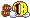• Replies 745
• Created

#### Popular Days

Ou de la pub, incription + 1 message + signature, mais c'est autorisé en signature.

##### Share on other sites

Je crois que google désactive les signatures de toutes façons##### Share on other sites

Quand j'ai vu ce message, je me suis posé la même question##### Share on other sites

• 2 weeks later...

Salut tout le monde je suis un nouveau « Inpactien » et comme vous j’aime bien la programmation et la création de site

( cf la signature).

J’ai une formation de comptable et donc les petits programmes que je construis sont dédiés à la comptabilité (entre autre).

J’ai choisi le PHP/MYSQL comme langage de programmation, car une fois conçu le programme peut être accessible par internet sans aucune condition.

Contrairement au Java script qui nécessite l’intégration d’une machine virtuelle.

Et vous quel est votre langage de programmation préféré ?

PS : Je suis à l’écoute des débutants qui voudraient créer correctement leur premier site web.

##### Share on other sites

• 3 weeks later...

moi je travailles en html avec webexpert 6

##### Share on other sites

• 2 weeks later...
• 2 weeks later...

Je viens de découvrir un truc assez marrant dans le code-source de Java, et plus exactement dans la classe Object : la méthode wait()

```public final void wait(long timeout, int nanos) throws InterruptedException {
if (timeout < 0) {
throw new IllegalArgumentException("timeout value is negative");
}

if (nanos < 0 || nanos > 999999) {
throw new IllegalArgumentException(
"nanosecond timeout value out of range");
}

if (nanos >= 500000 || (nanos != 0 && timeout == 0)) {
timeout++;
}

wait(timeout);
}```

Le truc se trouve dans le dernier 'if' et le 'return'... En gros, cette méthode en a à moitié rien à foutre des nanosecondes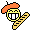##### Share on other sites

Seulement à moitié ?La doc ne dirait pas un truc du style "The time spent in the body of the method should roughly correspond to the amount of nanoseconds provided." tant qu'à faire ?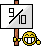##### Share on other sites

• 1 month later...

Lu tout le monde, j'ai un petit probleme au niveau de mon logiciel en console

c'est en C, merci d'avance...

Le smiley c'est "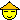"

##### Share on other sites

Merci d'utiliser les balises "code" ou en l'occurrence "codebox" vu la longueur de ton code.

D'autre part, comprend que ton message ne donne pas vraiment envie de t'aide. Pour schématiser : je copie-colle du code et je rajoute "Pourquoi ça marche pas" (note que j'ai traduis)

Essaies au moins d'introduire la chose et donner plus d'infos.

Je t'invite donc à corriger ton post en conséquence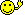##### Share on other sites

C'est un logiciel qui genere des nombres au hazard pour la roulette de casino

J'ai tout bien taper mais il y a une erreur inconnu :(

```#include <stdio.h>
#include <stdlib.h>
#include <time.h>

long genereHaz()
{
long nb = 0;
const long MAX = 36, MIN = 0;
srang(time(NULL));
nb = (rand() % (MAX - MIN + 1)) + MIN;
return nb;
}

int main(int argc, char *argv[])
{
long serie = 0;
printf("Generateur de series aléatoire");
printf("\n\t\t\tby K-1!BR3");
printf("\n\nCombien de nombres aleatoire veus-tu ?\n");
scanf("%ld", &serie);
long compteur = 0;
printf("\n");
for (compteur = 0 ; compteur < serie ; compteur++)
{

long nb = genereHaz();
if (nb = 0)
{
printf("\n0  Vert");
FILE* fichier = NULL;
fichier = fopen("Generateur de series/serie.txt", "w");
if (fichier != NULL)
{
fputs("\n0  Vert", fichier);
fclose(fichier);
}
else
printf("\nerreur d'ouvertur du fichier, le dossier dans lequel se trouve l'appliquation doit s'appeler Generateur de series\n\n");
}

else if (nb = 1)
{
printf("\n0  Vert");
FILE* fichier = NULL;
fichier = fopen("Generateur de series/serie.txt", "w");
if (fichier != NULL)
{
fputs("\n0  Vert", fichier);
fclose(fichier);
}
else
printf("\nerreur d'ouvertur du fichier, le dossier dans lequel se trouve l'appliquation doit s'appeler Generateur de series\n\n");
}

else if (nb = 2)
{
printf("\n0  Vert");
FILE* fichier = NULL;
fichier = fopen("Generateur de series/serie.txt", "w");
if (fichier != NULL)
{
fputs("\n0  Vert", fichier);
fclose(fichier);
}
else
printf("\nerreur d'ouvertur du fichier, le dossier dans lequel se trouve l'appliquation doit s'appeler Generateur de series\n\n");
}

else if (nb = 3)
{
printf("\n0  Vert");
FILE* fichier = NULL;
fichier = fopen("Generateur de series/serie.txt", "w");
if (fichier != NULL)
{
fputs("\n0  Vert", fichier);
fclose(fichier);
}
else
printf("\nerreur d'ouvertur du fichier, le dossier dans lequel se trouve l'appliquation doit s'appeler Generateur de series\n\n");
}

else if (nb = 4)
{
printf("\n0  Vert");
FILE* fichier = NULL;
fichier = fopen("Generateur de series/serie.txt", "w");
if (fichier != NULL)
{
fputs("\n0  Vert", fichier);
fclose(fichier);
}
else
printf("\nerreur d'ouvertur du fichier, le dossier dans lequel se trouve l'appliquation doit s'appeler Generateur de series\n\n");
}

else if (nb = 5)
{
printf("\n0  Vert");
FILE* fichier = NULL;
fichier = fopen("Generateur de series/serie.txt", "w");
if (fichier != NULL)
{
fputs("\n0  Vert", fichier);
fclose(fichier);
}
else
printf("\nerreur d'ouvertur du fichier, le dossier dans lequel se trouve l'appliquation doit s'appeler Generateur de series\n\n");
}

else if (nb = 6)
{
printf("\n0  Vert");
FILE* fichier = NULL;
fichier = fopen("Generateur de series/serie.txt", "w");
if (fichier != NULL)
{
fputs("\n0  Vert", fichier);
fclose(fichier);
}
else
printf("\nerreur d'ouvertur du fichier, le dossier dans lequel se trouve l'appliquation doit s'appeler Generateur de series\n\n");
}

else if (nb = 7)
{
printf("\n0  Vert");
FILE* fichier = NULL;
fichier = fopen("Generateur de series/serie.txt", "w");
if (fichier != NULL)
{
fputs("\n0  Vert", fichier);
fclose(fichier);
}
else
printf("\nerreur d'ouvertur du fichier, le dossier dans lequel se trouve l'appliquation doit s'appeler Generateur de series\n\n");
}

else if (nb =
{
printf("\n0  Vert");
FILE* fichier = NULL;
fichier = fopen("Generateur de series/serie.txt", "w");
if (fichier != NULL)
{
fputs("\n0  Vert", fichier);
fclose(fichier);
}
else
printf("\nerreur d'ouvertur du fichier, le dossier dans lequel se trouve l'appliquation doit s'appeler Generateur de series\n\n");
}

else if (nb = 9)
{
printf("\n0  Vert");
FILE* fichier = NULL;
fichier = fopen("Generateur de series/serie.txt", "w");
if (fichier != NULL)
{
fputs("\n0  Vert", fichier);
fclose(fichier);
}
else
printf("\nerreur d'ouvertur du fichier, le dossier dans lequel se trouve l'appliquation doit s'appeler Generateur de series\n\n");
}

else if (nb = 10)
{
printf("\n0  Vert");
FILE* fichier = NULL;
fichier = fopen("Generateur de series/serie.txt", "w");
if (fichier != NULL)
{
fputs("\n0  Vert", fichier);
fclose(fichier);
}
else
printf("\nerreur d'ouvertur du fichier, le dossier dans lequel se trouve l'appliquation doit s'appeler Generateur de series\n\n");
}

else if (nb = 11)
{
printf("\n0  Vert");
FILE* fichier = NULL;
fichier = fopen("Generateur de series/serie.txt", "w");
if (fichier != NULL)
{
fputs("\n0  Vert", fichier);
fclose(fichier);
}
else
printf("\nerreur d'ouvertur du fichier, le dossier dans lequel se trouve l'appliquation doit s'appeler Generateur de series\n\n");
}

else if (nb = 12)
{
printf("\n0  Vert");
FILE* fichier = NULL;
fichier = fopen("Generateur de series/serie.txt", "w");
if (fichier != NULL)
{
fputs("\n0  Vert", fichier);
fclose(fichier);
}
else
printf("\nerreur d'ouvertur du fichier, le dossier dans lequel se trouve l'appliquation doit s'appeler Generateur de series\n\n");
}

else if (nb = 13)
{
printf("\n0  Vert");
FILE* fichier = NULL;
fichier = fopen("Generateur de series/serie.txt", "w");
if (fichier != NULL)
{
fputs("\n0  Vert", fichier);
fclose(fichier);
}
else
printf("\nerreur d'ouvertur du fichier, le dossier dans lequel se trouve l'appliquation doit s'appeler Generateur de series\n\n");
}

else if (nb = 14)
{
printf("\n0  Vert");
FILE* fichier = NULL;
fichier = fopen("Generateur de series/serie.txt", "w");
if (fichier != NULL)
{
fputs("\n0  Vert", fichier);
fclose(fichier);
}
else
printf("\nerreur d'ouvertur du fichier, le dossier dans lequel se trouve l'appliquation doit s'appeler Generateur de series\n\n");
}

else if (nb = 15)
{
printf("\n0  Vert");
FILE* fichier = NULL;
fichier = fopen("Generateur de series/serie.txt", "w");
if (fichier != NULL)
{
fputs("\n0  Vert", fichier);
fclose(fichier);
}
else
printf("\nerreur d'ouvertur du fichier, le dossier dans lequel se trouve l'appliquation doit s'appeler Generateur de series\n\n");
}

else if (nb = 16)
{
printf("\n0  Vert");
FILE* fichier = NULL;
fichier = fopen("Generateur de series/serie.txt", "w");
if (fichier != NULL)
{
fputs("\n0  Vert", fichier);
fclose(fichier);
}
else
printf("\nerreur d'ouvertur du fichier, le dossier dans lequel se trouve l'appliquation doit s'appeler Generateur de series\n\n");
}

else if (nb = 16)
{
printf("\n0  Vert");
FILE* fichier = NULL;
fichier = fopen("Generateur de series/serie.txt", "w");
if (fichier != NULL)
{
fputs("\n0  Vert", fichier);
fclose(fichier);
}
else
printf("\nerreur d'ouvertur du fichier, le dossier dans lequel se trouve l'appliquation doit s'appeler Generateur de series\n\n");
}

else if (nb = 17)
{
printf("\n0  Vert");
FILE* fichier = NULL;
fichier = fopen("Generateur de series/serie.txt", "w");
if (fichier != NULL)
{
fputs("\n0  Vert", fichier);
fclose(fichier);
}
else
printf("\nerreur d'ouvertur du fichier, le dossier dans lequel se trouve l'appliquation doit s'appeler Generateur de series\n\n");
}

else if (nb = 18)
{
printf("\n0  Vert");
FILE* fichier = NULL;
fichier = fopen("Generateur de series/serie.txt", "w");
if (fichier != NULL)
{
fputs("\n0  Vert", fichier);
fclose(fichier);
}
else
printf("\nerreur d'ouvertur du fichier, le dossier dans lequel se trouve l'appliquation doit s'appeler Generateur de series\n\n");
}

else if (nb = 19)
{
printf("\n0  Vert");
FILE* fichier = NULL;
fichier = fopen("Generateur de series/serie.txt", "w");
if (fichier != NULL)
{
fputs("\n0  Vert", fichier);
fclose(fichier);
}
else
printf("\nerreur d'ouvertur du fichier, le dossier dans lequel se trouve l'appliquation doit s'appeler Generateur de series\n\n");
}

else if (nb = 20)
{
printf("\n0  Vert");
FILE* fichier = NULL;
fichier = fopen("Generateur de series/serie.txt", "w");
if (fichier != NULL)
{
fputs("\n0  Vert", fichier);
fclose(fichier);
}
else
printf("\nerreur d'ouvertur du fichier, le dossier dans lequel se trouve l'appliquation doit s'appeler Generateur de series\n\n");
}

else if (nb = 21)
{
printf("\n0  Vert");
FILE* fichier = NULL;
fichier = fopen("Generateur de series/serie.txt", "w");
if (fichier != NULL)
{
fputs("\n0  Vert", fichier);
fclose(fichier);
}
else
printf("\nerreur d'ouvertur du fichier, le dossier dans lequel se trouve l'appliquation doit s'appeler Generateur de series\n\n");
}

else if (nb = 22)
{
printf("\n0  Vert");
FILE* fichier = NULL;
fichier = fopen("Generateur de series/serie.txt", "w");
if (fichier != NULL)
{
fputs("\n0  Vert", fichier);
fclose(fichier);
}
else
printf("\nerreur d'ouvertur du fichier, le dossier dans lequel se trouve l'appliquation doit s'appeler Generateur de series\n\n");
}

else if (nb = 23)
{
printf("\n0  Vert");
FILE* fichier = NULL;
fichier = fopen("Generateur de series/serie.txt", "w");
if (fichier != NULL)
{
fputs("\n0  Vert", fichier);
fclose(fichier);
}
else
printf("\nerreur d'ouvertur du fichier, le dossier dans lequel se trouve l'appliquation doit s'appeler Generateur de series\n\n");
}

else if (nb = 24)
{
printf("\n0  Vert");
FILE* fichier = NULL;
fichier = fopen("Generateur de series/serie.txt", "w");
if (fichier != NULL)
{
fputs("\n0  Vert", fichier);
fclose(fichier);
}
else
printf("\nerreur d'ouvertur du fichier, le dossier dans lequel se trouve l'appliquation doit s'appeler Generateur de series\n\n");
}

else if (nb = 25)
{
printf("\n0  Vert");
FILE* fichier = NULL;
fichier = fopen("Generateur de series/serie.txt", "w");
if (fichier != NULL)
{
fputs("\n0  Vert", fichier);
fclose(fichier);
}
else
printf("\nerreur d'ouvertur du fichier, le dossier dans lequel se trouve l'appliquation doit s'appeler Generateur de series\n\n");
}

else if (nb = 26)
{
printf("\n0  Vert");
FILE* fichier = NULL;
fichier = fopen("Generateur de series/serie.txt", "w");
if (fichier != NULL)
{
fputs("\n0  Vert", fichier);
fclose(fichier);
}
else
printf("\nerreur d'ouvertur du fichier, le dossier dans lequel se trouve l'appliquation doit s'appeler Generateur de series\n\n");
}

else if (nb = 27)
{
printf("\n0  Vert");
FILE* fichier = NULL;
fichier = fopen("Generateur de series/serie.txt", "w");
if (fichier != NULL)
{
fputs("\n0  Vert", fichier);
fclose(fichier);
}
else
printf("\nerreur d'ouvertur du fichier, le dossier dans lequel se trouve l'appliquation doit s'appeler Generateur de series\n\n");
}

else if (nb = 28)
{
printf("\n0  Vert");
FILE* fichier = NULL;
fichier = fopen("Generateur de series/serie.txt", "w");
if (fichier != NULL)
{
fputs("\n0  Vert", fichier);
fclose(fichier);
}
else
printf("\nerreur d'ouvertur du fichier, le dossier dans lequel se trouve l'appliquation doit s'appeler Generateur de series\n\n");
}

else if (nb = 29)
{
printf("\n0  Vert");
FILE* fichier = NULL;
fichier = fopen("Generateur de series/serie.txt", "w");
if (fichier != NULL)
{
fputs("\n0  Vert", fichier);
fclose(fichier);
}
else
printf("\nerreur d'ouvertur du fichier, le dossier dans lequel se trouve l'appliquation doit s'appeler Generateur de series\n\n");
}

else if (nb = 30)
{
printf("\n0  Vert");
FILE* fichier = NULL;
fichier = fopen("Generateur de series/serie.txt", "w");
if (fichier != NULL)
{
fputs("\n0  Vert", fichier);
fclose(fichier);
}
else
printf("\nerreur d'ouvertur du fichier, le dossier dans lequel se trouve l'appliquation doit s'appeler Generateur de series\n\n");
}

else if (nb = 31)
{
printf("\n0  Vert");
FILE* fichier = NULL;
fichier = fopen("Generateur de series/serie.txt", "w");
if (fichier != NULL)
{
fputs("\n0  Vert", fichier);
fclose(fichier);
}
else
printf("\nerreur d'ouvertur du fichier, le dossier dans lequel se trouve l'appliquation doit s'appeler Generateur de series\n\n");
}

else if (nb = 32)
{
printf("\n0  Vert");
FILE* fichier = NULL;
fichier = fopen("Generateur de series/serie.txt", "w");
if (fichier != NULL)
{
fputs("\n0  Vert", fichier);
fclose(fichier);
}
else
printf("\nerreur d'ouvertur du fichier, le dossier dans lequel se trouve l'appliquation doit s'appeler Generateur de series\n\n");
}

else if (nb = 33)
{
printf("\n0  Vert");
FILE* fichier = NULL;
fichier = fopen("Generateur de series/serie.txt", "w");
if (fichier != NULL)
{
fputs("\n0  Vert", fichier);
fclose(fichier);
}
else
printf("\nerreur d'ouvertur du fichier, le dossier dans lequel se trouve l'appliquation doit s'appeler Generateur de series\n\n");
}

else if (nb = 34)
{
printf("\n0  Vert");
FILE* fichier = NULL;
fichier = fopen("Generateur de series/serie.txt", "w");
if (fichier != NULL)
{
fputs("\n0  Vert", fichier);
fclose(fichier);
}
else
printf("\nerreur d'ouvertur du fichier, le dossier dans lequel se trouve l'appliquation doit s'appeler Generateur de series\n\n");
}

else if (nb = 35)
{
printf("\n0  Vert");
FILE* fichier = NULL;
fichier = fopen("Generateur de series/serie.txt", "w");
if (fichier != NULL)
{
fputs("\n0  Vert", fichier);
fclose(fichier);
}
else
printf("\nerreur d'ouvertur du fichier, le dossier dans lequel se trouve l'appliquation doit s'appeler Generateur de series\n\n");
}

else if (nb = 36)
{
printf("\n0  Vert");
FILE* fichier = NULL;
fichier = fopen("Generateur de series/serie.txt", "w");
if (fichier != NULL)
{
fputs("\n0  Vert", fichier);
fclose(fichier);
}
else
printf("\nerreur d'ouvertur du fichier, le dossier dans lequel se trouve l'appliquation doit s'appeler Generateur de series\n\n");
}

}

printf("\nUn bloc note contenant la serie a ete cree dans le dossier Generateur de series\n\n");
system("PAUSE");
return 0;
}
```

dsl quarky

##### Share on other sites

voici l'erreur

C:\Documents and Settings\...\Bureau\Generateur de series\Makefile.win [build Error] [Projet1.exe] Error 1

upup

##### Share on other sites

On n'arrivera à rien avec un tel message d'erreur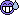il faudrait un truc plus détaillé (log de génération ou autre)

Tu utilise quel outil pour compiler ?

##### Share on other sites

j'ai rien d'autre :(

dev c++

##### Share on other sites

Salut,

Je ne connais pas la fonction srang mais srand, celle-là je la connais.

Une condition "si égal" ne s'écrit pas "if (nb = 10)" mais "if (nb == 10)".

Un switch case pourrait être mieux, non?

Ne veux tu pas ajouter à la suite de ton fichier texte? Si c'est le cas, essaye les option "a+" plutôt que "w" dans tes fopen.

Cordialement,

Barroud.

##### Share on other sites

Merci infiniment barroud pour tout ces conseils, mon log fonctionne correctement maintenant ^^ t'es plutôt doué...

Mise à part que il y a un erreur d'ouverture du .txt :(

```#include
#include
#include

long genereHaz()
{
long nb = 0;
const long MAX = 36, MIN = 0;
srand(time(NULL));
nb = (rand() % (MAX - MIN + 1)) + MIN;
return nb;
}

int main(int argc, char *argv[])
{
long serie = 0;
printf("Generateur de series aleatoire");
printf("\n\t\t\tby K-1!BR3");
printf("\n\nCombien de nombres aleatoire veus-tu ?\n");
scanf("%ld", &serie);
long compteur = 0;
printf("\n");
for (compteur = 0 ; compteur < serie ; compteur++)
{

long nb = genereHaz();
if (nb == 0)
{
printf("\n0  Vert");
FILE* fichier = NULL;
fichier = fopen("Generateur de series/serie.txt", "a+");
if (fichier != NULL)
{
fputs("\n0  Vert", fichier);
fclose(fichier);
}
else
printf("\nerreur d'ouvertur du fichier, le dossier dans lequel se trouve l'appliquation doit s'appeler Generateur de series\n\n");
}

else if (nb == 1)
{
printf("\n0  Vert");
FILE* fichier = NULL;
fichier = fopen("Generateur de series/serie.txt", "a+");
if (fichier != NULL)
{
fputs("\n0  Vert", fichier);
fclose(fichier);
}
else
printf("\nerreur d'ouvertur du fichier, le dossier dans lequel se trouve l'appliquation doit s'appeler Generateur de series\n\n");
}

else if (nb == 2)
{
printf("\n0  Vert");
FILE* fichier = NULL;
fichier = fopen("Generateur de series/serie.txt", "a+");
if (fichier != NULL)
{
fputs("\n0  Vert", fichier);
fclose(fichier);
}
else
printf("\nerreur d'ouvertur du fichier, le dossier dans lequel se trouve l'appliquation doit s'appeler Generateur de series\n\n");
}

else if (nb == 3)
{
printf("\n0  Vert");
FILE* fichier = NULL;
fichier = fopen("Generateur de series/serie.txt", "a+");
if (fichier != NULL)
{
fputs("\n0  Vert", fichier);
fclose(fichier);
}
else
printf("\nerreur d'ouvertur du fichier, le dossier dans lequel se trouve l'appliquation doit s'appeler Generateur de series\n\n");
}

else if (nb == 4)
{
printf("\n0  Vert");
FILE* fichier = NULL;
fichier = fopen("Generateur de series/serie.txt", "a+");
if (fichier != NULL)
{
fputs("\n0  Vert", fichier);
fclose(fichier);
}
else
printf("\nerreur d'ouvertur du fichier, le dossier dans lequel se trouve l'appliquation doit s'appeler Generateur de series\n\n");
}

else if (nb == 5)
{
printf("\n0  Vert");
FILE* fichier = NULL;
fichier = fopen("Generateur de series/serie.txt", "a+");
if (fichier != NULL)
{
fputs("\n0  Vert", fichier);
fclose(fichier);
}
else
printf("\nerreur d'ouvertur du fichier, le dossier dans lequel se trouve l'appliquation doit s'appeler Generateur de series\n\n");
}

else if (nb == 6)
{
printf("\n0  Vert");
FILE* fichier = NULL;
fichier = fopen("Generateur de series/serie.txt", "a+");
if (fichier != NULL)
{
fputs("\n0  Vert", fichier);
fclose(fichier);
}
else
printf("\nerreur d'ouvertur du fichier, le dossier dans lequel se trouve l'appliquation doit s'appeler Generateur de series\n\n");
}

else if (nb == 7)
{
printf("\n0  Vert");
FILE* fichier = NULL;
fichier = fopen("Generateur de series/serie.txt", "a+");
if (fichier != NULL)
{
fputs("\n0  Vert", fichier);
fclose(fichier);
}
else
printf("\nerreur d'ouvertur du fichier, le dossier dans lequel se trouve l'appliquation doit s'appeler Generateur de series\n\n");
}

else if (nb ==
{
printf("\n0  Vert");
FILE* fichier = NULL;
fichier = fopen("Generateur de series/serie.txt", "a+");
if (fichier != NULL)
{
fputs("\n0  Vert", fichier);
fclose(fichier);
}
else
printf("\nerreur d'ouvertur du fichier, le dossier dans lequel se trouve l'appliquation doit s'appeler Generateur de series\n\n");
}

else if (nb == 9)
{
printf("\n0  Vert");
FILE* fichier = NULL;
fichier = fopen("Generateur de series/serie.txt", "a+");
if (fichier != NULL)
{
fputs("\n0  Vert", fichier);
fclose(fichier);
}
else
printf("\nerreur d'ouvertur du fichier, le dossier dans lequel se trouve l'appliquation doit s'appeler Generateur de series\n\n");
}

else if (nb == 10)
{
printf("\n0  Vert");
FILE* fichier = NULL;
fichier = fopen("Generateur de series/serie.txt", "a+");
if (fichier != NULL)
{
fputs("\n0  Vert", fichier);
fclose(fichier);
}
else
printf("\nerreur d'ouvertur du fichier, le dossier dans lequel se trouve l'appliquation doit s'appeler Generateur de series\n\n");
}

else if (nb == 11)
{
printf("\n0  Vert");
FILE* fichier = NULL;
fichier = fopen("Generateur de series/serie.txt", "a+");
if (fichier != NULL)
{
fputs("\n0  Vert", fichier);
fclose(fichier);
}
else
printf("\nerreur d'ouvertur du fichier, le dossier dans lequel se trouve l'appliquation doit s'appeler Generateur de series\n\n");
}

else if (nb == 12)
{
printf("\n0  Vert");
FILE* fichier = NULL;
fichier = fopen("Generateur de series/serie.txt", "a+");
if (fichier != NULL)
{
fputs("\n0  Vert", fichier);
fclose(fichier);
}
else
printf("\nerreur d'ouvertur du fichier, le dossier dans lequel se trouve l'appliquation doit s'appeler Generateur de series\n\n");
}

else if (nb == 13)
{
printf("\n0  Vert");
FILE* fichier = NULL;
fichier = fopen("Generateur de series/serie.txt", "a+");
if (fichier != NULL)
{
fputs("\n0  Vert", fichier);
fclose(fichier);
}
else
printf("\nerreur d'ouvertur du fichier, le dossier dans lequel se trouve l'appliquation doit s'appeler Generateur de series\n\n");
}

else if (nb == 14)
{
printf("\n0  Vert");
FILE* fichier = NULL;
fichier = fopen("Generateur de series/serie.txt", "a+");
if (fichier != NULL)
{
fputs("\n0  Vert", fichier);
fclose(fichier);
}
else
printf("\nerreur d'ouvertur du fichier, le dossier dans lequel se trouve l'appliquation doit s'appeler Generateur de series\n\n");
}

else if (nb == 15)
{
printf("\n0  Vert");
FILE* fichier = NULL;
fichier = fopen("Generateur de series/serie.txt", "a+");
if (fichier != NULL)
{
fputs("\n0  Vert", fichier);
fclose(fichier);
}
else
printf("\nerreur d'ouvertur du fichier, le dossier dans lequel se trouve l'appliquation doit s'appeler Generateur de series\n\n");
}

else if (nb == 16)
{
printf("\n0  Vert");
FILE* fichier = NULL;
fichier = fopen("Generateur de series/serie.txt", "a+");
if (fichier != NULL)
{
fputs("\n0  Vert", fichier);
fclose(fichier);
}
else
printf("\nerreur d'ouvertur du fichier, le dossier dans lequel se trouve l'appliquation doit s'appeler Generateur de series\n\n");
}

else if (nb == 16)
{
printf("\n0  Vert");
FILE* fichier = NULL;
fichier = fopen("Generateur de series/serie.txt", "a+");
if (fichier != NULL)
{
fputs("\n0  Vert", fichier);
fclose(fichier);
}
else
printf("\nerreur d'ouvertur du fichier, le dossier dans lequel se trouve l'appliquation doit s'appeler Generateur de series\n\n");
}

else if (nb == 17)
{
printf("\n0  Vert");
FILE* fichier = NULL;
fichier = fopen("Generateur de series/serie.txt", "a+");
if (fichier != NULL)
{
fputs("\n0  Vert", fichier);
fclose(fichier);
}
else
printf("\nerreur d'ouvertur du fichier, le dossier dans lequel se trouve l'appliquation doit s'appeler Generateur de series\n\n");
}

else if (nb == 18)
{
printf("\n0  Vert");
FILE* fichier = NULL;
fichier = fopen("Generateur de series/serie.txt", "a+");
if (fichier != NULL)
{
fputs("\n0  Vert", fichier);
fclose(fichier);
}
else
printf("\nerreur d'ouvertur du fichier, le dossier dans lequel se trouve l'appliquation doit s'appeler Generateur de series\n\n");
}

else if (nb == 19)
{
printf("\n0  Vert");
FILE* fichier = NULL;
fichier = fopen("Generateur de series/serie.txt", "a+");
if (fichier != NULL)
{
fputs("\n0  Vert", fichier);
fclose(fichier);
}
else
printf("\nerreur d'ouvertur du fichier, le dossier dans lequel se trouve l'appliquation doit s'appeler Generateur de series\n\n");
}

else if (nb == 20)
{
printf("\n0  Vert");
FILE* fichier = NULL;
fichier = fopen("Generateur de series/serie.txt", "a+");
if (fichier != NULL)
{
fputs("\n0  Vert", fichier);
fclose(fichier);
}
else
printf("\nerreur d'ouvertur du fichier, le dossier dans lequel se trouve l'appliquation doit s'appeler Generateur de series\n\n");
}

else if (nb == 21)
{
printf("\n0  Vert");
FILE* fichier = NULL;
fichier = fopen("Generateur de series/serie.txt", "a+");
if (fichier != NULL)
{
fputs("\n0  Vert", fichier);
fclose(fichier);
}
else
printf("\nerreur d'ouvertur du fichier, le dossier dans lequel se trouve l'appliquation doit s'appeler Generateur de series\n\n");
}

else if (nb == 22)
{
printf("\n0  Vert");
FILE* fichier = NULL;
fichier = fopen("Generateur de series/serie.txt", "a+");
if (fichier != NULL)
{
fputs("\n0  Vert", fichier);
fclose(fichier);
}
else
printf("\nerreur d'ouvertur du fichier, le dossier dans lequel se trouve l'appliquation doit s'appeler Generateur de series\n\n");
}

else if (nb == 23)
{
printf("\n0  Vert");
FILE* fichier = NULL;
fichier = fopen("Generateur de series/serie.txt", "a+");
if (fichier != NULL)
{
fputs("\n0  Vert", fichier);
fclose(fichier);
}
else
printf("\nerreur d'ouvertur du fichier, le dossier dans lequel se trouve l'appliquation doit s'appeler Generateur de series\n\n");
}

else if (nb == 24)
{
printf("\n0  Vert");
FILE* fichier = NULL;
fichier = fopen("Generateur de series/serie.txt", "a+");
if (fichier != NULL)
{
fputs("\n0  Vert", fichier);
fclose(fichier);
}
else
printf("\nerreur d'ouvertur du fichier, le dossier dans lequel se trouve l'appliquation doit s'appeler Generateur de series\n\n");
}

else if (nb == 25)
{
printf("\n0  Vert");
FILE* fichier = NULL;
fichier = fopen("Generateur de series/serie.txt", "a+");
if (fichier != NULL)
{
fputs("\n0  Vert", fichier);
fclose(fichier);
}
else
printf("\nerreur d'ouvertur du fichier, le dossier dans lequel se trouve l'appliquation doit s'appeler Generateur de series\n\n");
}

else if (nb == 26)
{
printf("\n0  Vert");
FILE* fichier = NULL;
fichier = fopen("Generateur de series/serie.txt", "a+");
if (fichier != NULL)
{
fputs("\n0  Vert", fichier);
fclose(fichier);
}
else
printf("\nerreur d'ouvertur du fichier, le dossier dans lequel se trouve l'appliquation doit s'appeler Generateur de series\n\n");
}

else if (nb == 27)
{
printf("\n0  Vert");
FILE* fichier = NULL;
fichier = fopen("Generateur de series/serie.txt", "a+");
if (fichier != NULL)
{
fputs("\n0  Vert", fichier);
fclose(fichier);
}
else
printf("\nerreur d'ouvertur du fichier, le dossier dans lequel se trouve l'appliquation doit s'appeler Generateur de series\n\n");
}

else if (nb == 28)
{
printf("\n0  Vert");
FILE* fichier = NULL;
fichier = fopen("Generateur de series/serie.txt", "a+");
if (fichier != NULL)
{
fputs("\n0  Vert", fichier);
fclose(fichier);
}
else
printf("\nerreur d'ouvertur du fichier, le dossier dans lequel se trouve l'appliquation doit s'appeler Generateur de series\n\n");
}

else if (nb == 29)
{
printf("\n0  Vert");
FILE* fichier = NULL;
fichier = fopen("Generateur de series/serie.txt", "a+");
if (fichier != NULL)
{
fputs("\n0  Vert", fichier);
fclose(fichier);
}
else
printf("\nerreur d'ouvertur du fichier, le dossier dans lequel se trouve l'appliquation doit s'appeler Generateur de series\n\n");
}

else if (nb == 30)
{
printf("\n0  Vert");
FILE* fichier = NULL;
fichier = fopen("Generateur de series/serie.txt", "a+");
if (fichier != NULL)
{
fputs("\n0  Vert", fichier);
fclose(fichier);
}
else
printf("\nerreur d'ouvertur du fichier, le dossier dans lequel se trouve l'appliquation doit s'appeler Generateur de series\n\n");
}

else if (nb == 31)
{
printf("\n0  Vert");
FILE* fichier = NULL;
fichier = fopen("Generateur de series/serie.txt", "a+");
if (fichier != NULL)
{
fputs("\n0  Vert", fichier);
fclose(fichier);
}
else
printf("\nerreur d'ouvertur du fichier, le dossier dans lequel se trouve l'appliquation doit s'appeler Generateur de series\n\n");
}

else if (nb == 32)
{
printf("\n0  Vert");
FILE* fichier = NULL;
fichier = fopen("Generateur de series/serie.txt", "a+");
if (fichier != NULL)
{
fputs("\n0  Vert", fichier);
fclose(fichier);
}
else
printf("\nerreur d'ouvertur du fichier, le dossier dans lequel se trouve l'appliquation doit s'appeler Generateur de series\n\n");
}

else if (nb == 33)
{
printf("\n0  Vert");
FILE* fichier = NULL;
fichier = fopen("Generateur de series/serie.txt", "a+");
if (fichier != NULL)
{
fputs("\n0  Vert", fichier);
fclose(fichier);
}
else
printf("\nerreur d'ouvertur du fichier, le dossier dans lequel se trouve l'appliquation doit s'appeler Generateur de series\n\n");
}

else if (nb == 34)
{
printf("\n0  Vert");
FILE* fichier = NULL;
fichier = fopen("Generateur de series/serie.txt", "a+");
if (fichier != NULL)
{
fputs("\n0  Vert", fichier);
fclose(fichier);
}
else
printf("\nerreur d'ouvertur du fichier, le dossier dans lequel se trouve l'appliquation doit s'appeler Generateur de series\n\n");
}

else if (nb == 35)
{
printf("\n0  Vert");
FILE* fichier = NULL;
fichier = fopen("Generateur de series/serie.txt", "a+");
if (fichier != NULL)
{
fputs("\n0  Vert", fichier);
fclose(fichier);
}
else
printf("\nerreur d'ouvertur du fichier, le dossier dans lequel se trouve l'appliquation doit s'appeler Generateur de series\n\n");
}

else if (nb == 36)
{
printf("\n0  Vert");
FILE* fichier = NULL;
fichier = fopen("Generateur de series/serie.txt", "a+");
if (fichier != NULL)
{
fputs("\n0  Vert", fichier);
fclose(fichier);
}
else
printf("\nerreur d'ouvertur du fichier, le dossier dans lequel se trouve l'appliquation doit s'appeler Generateur de series\n\n");
}

}

printf("\nUn bloc note contenant la serie a ete cree dans le dossier Generateur de series\n\n");
system("PAUSE");
return 0;
}
```

Pour le switch c'est vrai que sa aurai été mieux mais c'est pas grave je vais pas tout recommencer.

up

up

##### Share on other sites

Déjà tu peux voir quel est le problème en affichant le pourquoi de l'erreur. Par exemple :

```if (fichier != NULL)
{
// ...
}
else
{
printf(_strerror(NULL)); // Affiche le texte de description de la dernière erreur rencontrée par le programme
}```

##### Share on other sites

il y a un erreur d'ouverture du .txt :(

##### Share on other sites

Il est pas déjà ouvert ailleurs ?

Il existe bien au moment où tu veux l'ouvrir ?

##### Share on other sites

nn ya rien, il doit y avoir un bleme dans le les lignes de code mais je vois pas

##### Share on other sites

Tu ne nous aide pas beaucoup ^^

As-tu essayé la fonction que je t'ai montrée ? Si oui quel message affiche-t-elle ?

## Join the conversation

You can post now and register later. If you have an account, sign in now to post with your account.×   Pasted as rich text.   Paste as plain text instead

Only 75 emoji are allowed.